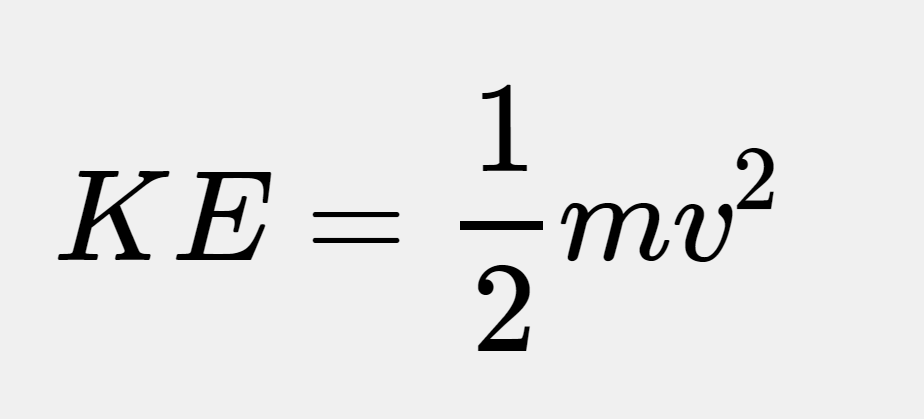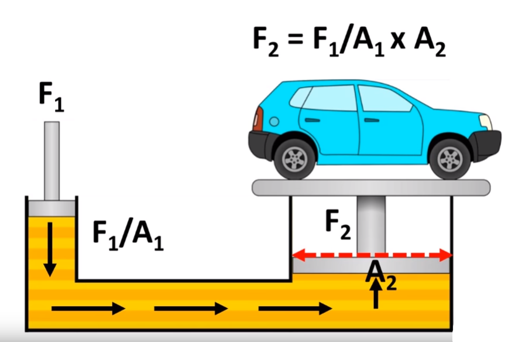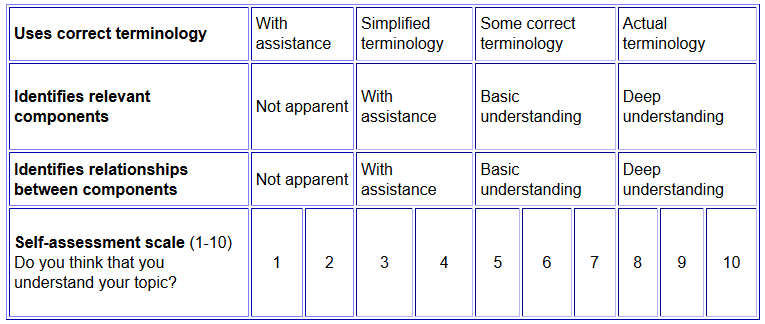# SILO 4.2 (DRAFT)

## Year 4, Term 2: Transportation

### Learning intention: Students explore common modes of transportation to develop an integrated understanding of forces, materials, energy and design factors.

NSW Syllabus
Australian Curriculum (version 9.0)
"A student investigates the suitability of natural and processed materials for a range of purposes." (ST2-7MW-T)
"Students learn to describe how forces and the properties of materials affect function in a product or system." (AC9TDE4K02)

## Week 1

Introduction to transportation

Ball bearings

Skateboards, roller skates and roller blades

Roller coasters

## Week 2

Conservation of energy

The law of conservation of energy states that energy can neither be created nor destroyed - only converted from one form of energy to another.  The following video (1:36) demonstrates this with vivid examples of kinetic and potential energy.

Kinetic energy

Kinetic energy is the energy that an object has because of its motion.  The formula for kinetic energy is:Kinetic Energy Calculator

## Week 3

Bicycles and gears

Gears were explained in SILO 4.1 'Simple machines' so the focus here will be on brakes and fixed-wheel bikes.

Newton's three laws of motion

Newton's three laws of motion are explained in the following video (3:32).  These laws were first stated by Isaac Newton in 1687.  The three laws may be summarised as follows:

1. Every object in a state of uniform motion will remain in that state of motion unless an external force acts on it.  This is also know as inertia.
2. Force equals mass times acceleration (F=ma)
3. For every action there is an equal and opposite reaction.

## Week 4

Hovercraftshttps://www.kiwico.com/diy/Science-Projects-for-Kids/3/project/Balloon-Hovercraft/2608

Rocket sled

## Week 5

Boats and buoyancy

Archimedes principle

Submarines

This video (1:56) from ABC news explains how nuclear-powered submarines work and how they compare to other types of submarines.  In light of the AUKUS alliance, working on nuclear submarines will require many more engineers worldwide, and particularly here in Australia.

## Week 6

Speed

Velocity

Acceleration

This video (2:02) explains how acceleration is the rate at which velocity changes over time.  It also features some examples of how to use mathematics to calculate the average acceleration.

## Week 7

Differentials

This video (5:18) is more advanced than Year 4 children would normally encounter but the main points are that wheels turn at different speeds when cornering and that gears are one way to enable this.  Students will also explore how wheels on a non-fixed axle such as on a pram or billy cart are free to turn at different rates.

Electric vehicles

Internal combustion engines

Motorcycles

Cars

## Week 8

Gliders

Aeroplanes

Rockets

Space ships

Air pressure experiments

The following video (10:18) is about straws and it explains that suction is really a difference in pressure.  It also makes the point that "Nothing sucks in science".

## Week 9

Frame of reference

Direction is relative as shown in this video (0:47) where clockwise and anti-clockwise depend on your frame of reference.

Relative motion

The following video (6:26) on relative motion is an important introduction to the idea that everything is in constant motion.  Related terms include 'frame of reference'.

Hydraulics

Pascal's principle states that the pressure applied to any part of an enclosed liquid will be transmitted equally in all directions through the liquid.  The following screenshot is from a video (3:22) about the application of Pascal's law in hydraulics.  It shows the formula for comparing both sides of a hydraulic jack.## Week 10

Moderated self-assessment